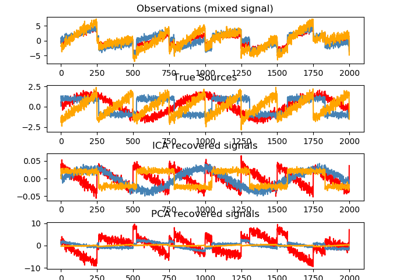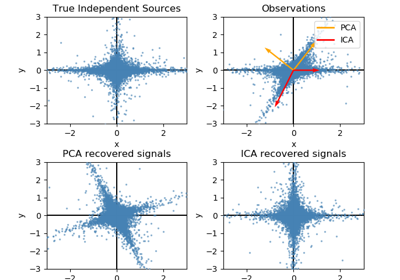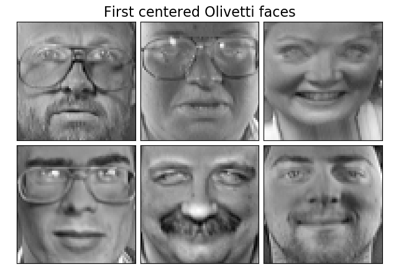# sklearn.decomposition.FastICA¶

class sklearn.decomposition.FastICA(n_components=None, algorithm='parallel', whiten=True, fun='logcosh', fun_args=None, max_iter=200, tol=0.0001, w_init=None, random_state=None)[source]

FastICA: a fast algorithm for Independent Component Analysis.

Read more in the User Guide.

Parameters: n_components : int, optional Number of components to use. If none is passed, all are used. algorithm : {‘parallel’, ‘deflation’} Apply parallel or deflational algorithm for FastICA. whiten : boolean, optional If whiten is false, the data is already considered to be whitened, and no whitening is performed. fun : string or function, optional. Default: ‘logcosh’ The functional form of the G function used in the approximation to neg-entropy. Could be either ‘logcosh’, ‘exp’, or ‘cube’. You can also provide your own function. It should return a tuple containing the value of the function, and of its derivative, in the point. Example: def my_g(x): return x ** 3, (3 * x ** 2).mean(axis=-1) fun_args : dictionary, optional Arguments to send to the functional form. If empty and if fun=’logcosh’, fun_args will take value {‘alpha’ : 1.0}. max_iter : int, optional Maximum number of iterations during fit. tol : float, optional Tolerance on update at each iteration. w_init : None of an (n_components, n_components) ndarray The mixing matrix to be used to initialize the algorithm. random_state : int, RandomState instance or None, optional (default=None) If int, random_state is the seed used by the random number generator; If RandomState instance, random_state is the random number generator; If None, the random number generator is the RandomState instance used by np.random. components_ : 2D array, shape (n_components, n_features) The unmixing matrix. mixing_ : array, shape (n_features, n_components) The mixing matrix. n_iter_ : int If the algorithm is “deflation”, n_iter is the maximum number of iterations run across all components. Else they are just the number of iterations taken to converge.

Notes

Implementation based on A. Hyvarinen and E. Oja, Independent Component Analysis: Algorithms and Applications, Neural Networks, 13(4-5), 2000, pp. 411-430

Examples

>>> from sklearn.datasets import load_digits
>>> from sklearn.decomposition import FastICA
>>> transformer = FastICA(n_components=7,
...         random_state=0)
>>> X_transformed = transformer.fit_transform(X)
>>> X_transformed.shape
(1797, 7)


Methods

 fit(X[, y]) Fit the model to X. fit_transform(X[, y]) Fit the model and recover the sources from X. get_params([deep]) Get parameters for this estimator. inverse_transform(X[, copy]) Transform the sources back to the mixed data (apply mixing matrix). set_params(**params) Set the parameters of this estimator. transform(X[, y, copy]) Recover the sources from X (apply the unmixing matrix).
__init__(n_components=None, algorithm='parallel', whiten=True, fun='logcosh', fun_args=None, max_iter=200, tol=0.0001, w_init=None, random_state=None)[source]
fit(X, y=None)[source]

Fit the model to X.

Parameters: X : array-like, shape (n_samples, n_features) Training data, where n_samples is the number of samples and n_features is the number of features. y : Ignored self
fit_transform(X, y=None)[source]

Fit the model and recover the sources from X.

Parameters: X : array-like, shape (n_samples, n_features) Training data, where n_samples is the number of samples and n_features is the number of features. y : Ignored X_new : array-like, shape (n_samples, n_components)
get_params(deep=True)[source]

Get parameters for this estimator.

Parameters: deep : boolean, optional If True, will return the parameters for this estimator and contained subobjects that are estimators. params : mapping of string to any Parameter names mapped to their values.
inverse_transform(X, copy=True)[source]

Transform the sources back to the mixed data (apply mixing matrix).

Parameters: X : array-like, shape (n_samples, n_components) Sources, where n_samples is the number of samples and n_components is the number of components. copy : bool (optional) If False, data passed to fit are overwritten. Defaults to True. X_new : array-like, shape (n_samples, n_features)
set_params(**params)[source]

Set the parameters of this estimator.

The method works on simple estimators as well as on nested objects (such as pipelines). The latter have parameters of the form <component>__<parameter> so that it’s possible to update each component of a nested object.

Returns: self
transform(X, y='deprecated', copy=True)[source]

Recover the sources from X (apply the unmixing matrix).

Parameters: X : array-like, shape (n_samples, n_features) Data to transform, where n_samples is the number of samples and n_features is the number of features. y : (ignored) Deprecated since version 0.19: This parameter will be removed in 0.21. copy : bool (optional) If False, data passed to fit are overwritten. Defaults to True. X_new : array-like, shape (n_samples, n_components)

## Examples using sklearn.decomposition.FastICA¶Blind source separation using FastICAFastICA on 2D point cloudsFaces dataset decompositions